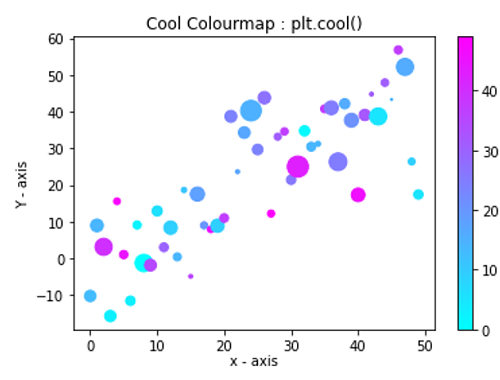# Python | matplotlib.pyplot.cool() for Cool Color Map

In this tutorial, we are going to learn how to change the color map to Cool color map in a figure?
Submitted by Anuj Singh, on August 06, 2020

There is an inbuilt defined function i.e. matplotlib.pyplot.cool(), using which we can switch the color map of the figure into a cool color map. The following example shows us how to switch color map into cool in python using matplotlib.

Illustration:## Python code for matplotlib.pyplot.cool() for cool color map

```import numpy as np
import matplotlib.pyplot as plt

data = {'a': np.arange(50),
'c': np.random.randint(0, 50, 50),
'd': np.random.randn(50)}
data['b'] = data['a'] + 10 * np.random.randn(50)
data['d'] = np.abs(data['d']) * 100

plt.scatter('a', 'b', c='c', s='d', data=data)
plt.ylabel('Y - axis')
plt.cool()
plt.title('Cool Colourmap : plt.cool()')
plt.colorbar()
plt.show()
```

Output:

```Output is as figure
```

Languages: » C » C++ » C++ STL » Java » Data Structure » C#.Net » Android » Kotlin » SQL
Web Technologies: » PHP » Python » JavaScript » CSS » Ajax » Node.js » Web programming/HTML
Solved programs: » C » C++ » DS » Java » C#
Aptitude que. & ans.: » C » C++ » Java » DBMS
Interview que. & ans.: » C » Embedded C » Java » SEO » HR
CS Subjects: » CS Basics » O.S. » Networks » DBMS » Embedded Systems » Cloud Computing
» Machine learning » CS Organizations » Linux » DOS
More: » Articles » Puzzles » News/Updates